Download Presentation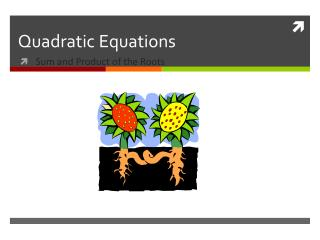# Quadratic Equations - PowerPoint PPT Presentation

Quadratic Equations. Sum and Product of the Roots. Aim: Use the sum and product of the roots in order to write a quadratic equation. Solve for x by using the quadratic formula:. a. What is the sum of its roots?. -3 + -4 =. -7. b. What is the product of its roots?.I am the owner, or an agent authorized to act on behalf of the owner, of the copyrighted work described.
Download PresentationAn Image/Link below is provided (as is) to download presentation

Download Policy: Content on the Website is provided to you AS IS for your information and personal use and may not be sold / licensed / shared on other websites without getting consent from its author.While downloading, if for some reason you are not able to download a presentation, the publisher may have deleted the file from their server.

- - - - - - - - - - - - - - - - - - - - - - - - - - E N D - - - - - - - - - - - - - - - - - - - - - - - - - -
Presentation Transcript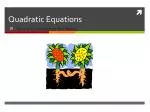• Sum and Product of the Roots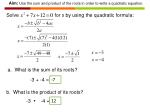Aim: Use the sum and product of the roots in order to write a quadratic equation.

Solve for x by using the quadratic formula:

a. What is the sum of its roots?

-3 + -4 =

-7

b. What is the product of its roots?

12

-3  -4 =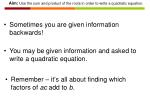Aim: Use the sum and product of the roots in order to write a quadratic equation.

• Sometimes you are given information backwards!
• You may be given information and asked to write a quadratic equation.
• Remember – it’s all about finding which factors of ac add to b.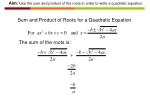Aim: Use the sum and product of the roots in order to write a quadratic equation.

Sum and Product of Roots for a Quadratic Equation

The sum of the roots is: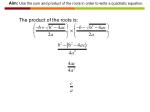Aim: Use the sum and product of the roots in order to write a quadratic equation.

The product of the roots is: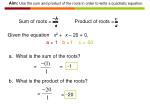Aim: Use the sum and product of the roots in order to write a quadratic equation.

Sum of roots = Product of roots =

Given the equation x2 + x – 20 = 0,

a =

1

b =

1

c =

-20

a. What is the sum of the roots?

=

=

-1

b. What is the product of the roots?

=

=

-20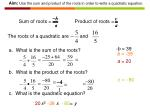Aim: Use the sum and product of the roots in order to write a quadratic equation.

Sum of roots = Product of roots =

The roots of a quadratic are and

-b = 39

a. What is the sum of the roots?

b = -39

a = 20

b. What is the product of the roots?

c = - 80

c. What is the quadratic equation?

20

x2

-39

x

- 80

= y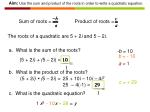Aim: Use the sum and product of the roots in order to write a quadratic equation.

Sum of roots = Product of roots =

The roots of a quadratic are 5 + 2i and 5 – 2i.

a. What is the sum of the roots?

-b = 10

b = - 10

(5 + 2i) + (5 – 2i) =

10

a = 1

b. What is the product of the roots?

(5 + 2i)  (5 – 2i) =

25 + 10i – 10i – 4i2

c = + 29

25 – 4(-1) =

29

c. What is the quadratic equation?

1

x2

- 10

x

+ 29

= y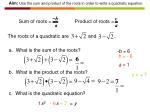Aim: Use the sum and product of the roots in order to write a quadratic equation.

Sum of roots = Product of roots =

The roots of a quadratic are and .

a. What is the sum of the roots?

-b = 6

b = - 6

a = 1

b. What is the product of the roots?

c = + 7

c. What is the quadratic equation?

1

x2

- 6

x

+ 7

= y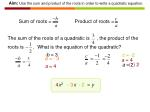Aim: Use the sum and product of the roots in order to write a quadratic equation.

Sum of roots = Product of roots =

The sum of the roots of a quadratic is , the product of the roots is . What is the equation of the quadratic?

-b = 3

c = -2

b = - 3

a = 4

( ) 2

a = 2

a = 4

4

x2

x

= y

- 3

- 2Aim: Use the sum and product of the roots in order to write a quadratic equation.

Sum of roots = Product of roots =

Find k such that -3 is a root of x2 + kx – 24 = 0.

Product =

Sum =